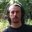Question-and-Answer Resource for the Building Energy Modeling Community
Get started with the Help page

# PMV-PPD Mistake in EnergyplusWhen I read the Pmv-Ppd values in the Energyplus program, I get values that do not fit the Fanger equation. For example, PMV is -0.3, PPD is 33% or PMV is -0.5, PPD is 38%. Do you think these results are correct?

edit retag close merge delete

through the calculate,I think it's wrong.the formula is PDD=100-95exp(-0.03353PMV^4-0.2179*PMV^2).but my PSS value isn't from the energyplus I did the calculations myself

Sort by » oldest newest most voted

Hi!

Could you provide more details about the problem?

I just checked with a simulation using the "RefBldgSmallOfficeNew2004_Chicago.idf" in the example files and "USA_IL_Chicago-OHare.Intl.AP.725300_TMY3.epw" weather file that comes with the EnergyPlus v9.4 installation.

I think the EnergyPlus calculated value for PPD agrees with the Fanger equation (same equation in the EnergyPlus code here) with the maximum percentage error being 21%. For a PMV value of -0.30006, the PPD by EnergyPlus was 6.87, and the error in PPD calculation was 2.15E-06% which is very small.

moreEnergyPlus PMV and PPD are right.

Just for information, they are referred to the previous calculation time step than reported. Therefore when reading simulaiton outputs, PMV/PPD values are calculated with reference to previous timestep MTA, MTR, VEL, RH, CLO and MET values

more